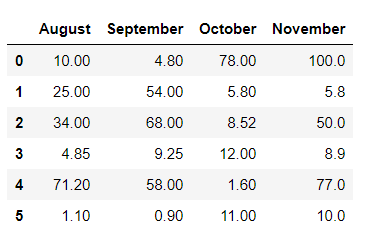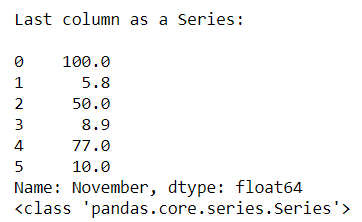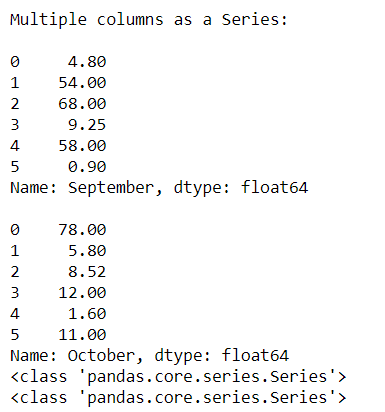# How to Convert Pandas DataFrame columns to a Series?

• Last Updated : 01 Oct, 2020

It is possible in pandas to convert columns of the pandas Data frame to series. Sometimes there is a need to converting columns of the data frame to another type like series for analyzing the data set.

Case 1: Converting the first column of the data frame to Series

Attention geek! Strengthen your foundations with the Python Programming Foundation Course and learn the basics.

To begin with, your interview preparations Enhance your Data Structures concepts with the Python DS Course. And to begin with your Machine Learning Journey, join the Machine Learning - Basic Level Course

## Python3

 `# Importing pandas module``import` `pandas as pd`` ` `# Creating a dictionary ``dit ``=` `{``'August'``: [``10``, ``25``, ``34``, ``4.85``, ``71.2``, ``1.1``], ``       ``'September'``: [``4.8``, ``54``, ``68``, ``9.25``, ``58``, ``0.9``], ``       ``'October'``: [``78``, ``5.8``, ``8.52``, ``12``, ``1.6``, ``11``], ``       ``'November'``: [``100``, ``5.8``, ``50``, ``8.9``, ``77``, ``10``] }`` ` `# Converting it to data frame``df ``=` `pd.DataFrame(data``=``dit)`` ` `# Original DataFrame``df`

Output:Converting the first column to series.

## Python3

 `# Converting first column i.e 'August' to Series``ser1 ``=` `df.ix[:,``0``]`` ` `print``(``"\n1st column as a Series:\n"``)``print``(ser1)`` ` `# Checking type``print``(``type``(ser1))`

Output:In the above example, we change the type of column ‘August‘ from the data frame to Series.

Case 2: Converting the last column of the data frame to Series

## Python3

 `# Importing pandas module``import` `pandas as pd`` ` `# Creating a dictionary ``dit ``=` `{``'August'``: [``10``, ``25``, ``34``, ``4.85``, ``71.2``, ``1.1``], ``       ``'September'``: [``4.8``, ``54``, ``68``, ``9.25``, ``58``, ``0.9``], ``       ``'October'``: [``78``, ``5.8``, ``8.52``, ``12``, ``1.6``, ``11``], ``       ``'November'``: [``100``, ``5.8``, ``50``, ``8.9``, ``77``, ``10``] }`` ` `# Converting it to data frame``df ``=` `pd.DataFrame(data``=``dit)`` ` `# Original DataFrame``df`

Output:Converting the last column to the series.

## Python3

 `# Converting last column i.e 'November' to Series``ser1 ``=` `df.ix[:,``3``]`` ` `print``(``"\nLast column as a Series:\n"``)``print``(ser1)`` ` `# Checking type``print``(``type``(ser1))`

Output:In the above example, we change the type of column ‘November‘ from the data frame to Series.

Case 3: Converting the multiple columns of the data frame to Series

## Python3

 `# Importing pandas module``import` `pandas as pd`` ` `# Creating a dictionary ``dit ``=` `{``'August'``: [``10``, ``25``, ``34``, ``4.85``, ``71.2``, ``1.1``], ``       ``'September'``: [``4.8``, ``54``, ``68``, ``9.25``, ``58``, ``0.9``], ``       ``'October'``: [``78``, ``5.8``, ``8.52``, ``12``, ``1.6``, ``11``], ``       ``'November'``: [``100``, ``5.8``, ``50``, ``8.9``, ``77``, ``10``] }`` ` `# Converting it to data frame``df ``=` `pd.DataFrame(data``=``dit)`` ` `# Original DataFrame``df`

Output:Converting multiple columns to series.

## Python3

 `# Converting multiple columns ``# i.e 'September' and 'October' to Series``ser1 ``=` `df.ix[:,``1``]``ser2 ``=` `df.ix[:,``2``]`` ` `print``(``"\nMultiple columns as a Series:\n"``)``print``(ser1)``print``()``print``(ser2)`` ` `# Checking type``print``(``type``(ser1))``print``(``type``(ser2))`

Output:In the above example, we change the type of 2 columns i.e ‘September‘ and ‘October’ from the data frame to Series.

My Personal Notes arrow_drop_up